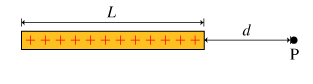# Problem: A straight wire of length L has a positive charge Q distributed along its length. Find the magnitude of the electric field due to the wire at a point located a distance d from one end of the wire along the line extending from the wire. Find Enet, the magnitude of the electric field at point P due to the total charge.Express your answer in terms of some or all of the variables Q, L, and d, and the constants k and/or ϵ0.

###### FREE Expert Solution

Electric field due to a small length of wire:

$\overline{){\mathbf{d}}{\mathbf{E}}{\mathbf{=}}{\mathbf{k}}\frac{\mathbf{d}\mathbf{Q}}{{\mathbf{x}}^{\mathbf{2}}}{\mathbf{=}}{\mathbf{k}}\frac{\mathbf{\lambda }\mathbf{d}\mathbf{x}}{{\mathbf{x}}^{\mathbf{2}}}}$

90% (64 ratings)###### Problem DetailsA straight wire of length L has a positive charge Q distributed along its length.

Find the magnitude of the electric field due to the wire at a point located a distance d from one end of the wire along the line extending from the wire. Find Enet, the magnitude of the electric field at point P due to the total charge.

Express your answer in terms of some or all of the variables Q, L, and d, and the constants k and/or ϵ0.